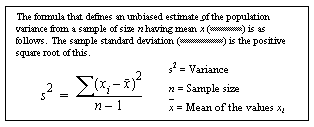# stddev_samp

Computes the standard deviation of a sample consisting of a numeric expression as a double. stdev and stddev are aliases for stddev_samp, and use the same syntax.

## Syntax

`stddev_samp ( [ all | distinct ] expression )`

## Parameters

• all – applies stddev_samp to all values. all is the default.
• distinct – eliminates duplicate values before stddev_samp is applied.
• expression – is any numeric datatype (float, real, or double precision) expression.

## Examples

• Example 1 – The following statement lists the average and standard deviation of the advances for each type of book in the pubs2 database.
```select type, avg(advance) as "avg",
where total_sales > 2000 group by type order by type```

## Usage

Computes the sample standard deviation of the provided value expression evaluated for each row of the group (if distinct was specified, then each row that remains after duplicates have been eliminated), defined as the square root of the sample variance.

Formula for Sample-Related Statistical Aggregate Functions